# Introduction

Here you will learn the basics of Alga, an implementation of an algebra of graphs. Every given examples is runnable, so please feel free to install alga (with `cabal` or `stack`) and have a GHCi console near you if you want to try the code. All you need to have is inside the `Algebra.Graph` module. Don’t hesitate to have a look at the module documentation if you want more informations.

If you encounter any bug (I hope you will not), please open an issue at https://github.com/snowleopard/alga/issues/.

# The graph definition

## The problem

Graphs are traditionally defined as a pair comprising a set V of vertices and a set E ⊆ V × V of edges. This is great when working with traditional imperative languages, but leads to some problems when trying to use it in a functional languages such as Haskell.

The idea of alga is to use an other definition of graph, more “functional-friendly“. As the most part of the “functional-friendly” data structures is recursive, such is the alga’s graph definition:

## A solution

``````data Graph a = Empty
| Vertex a
| Overlay (Graph a) (Graph a)
| Connect (Graph a) (Graph a)``````

So it says:

1. You have an only way to construct the empty graph, using the constructor `Empty` which does not take any argument.

2. You can construct a graph from anything, transforming it in a single vertex using the constructor `Vertex`.

3. You can overlay two graphs, that is just to put them next one to another.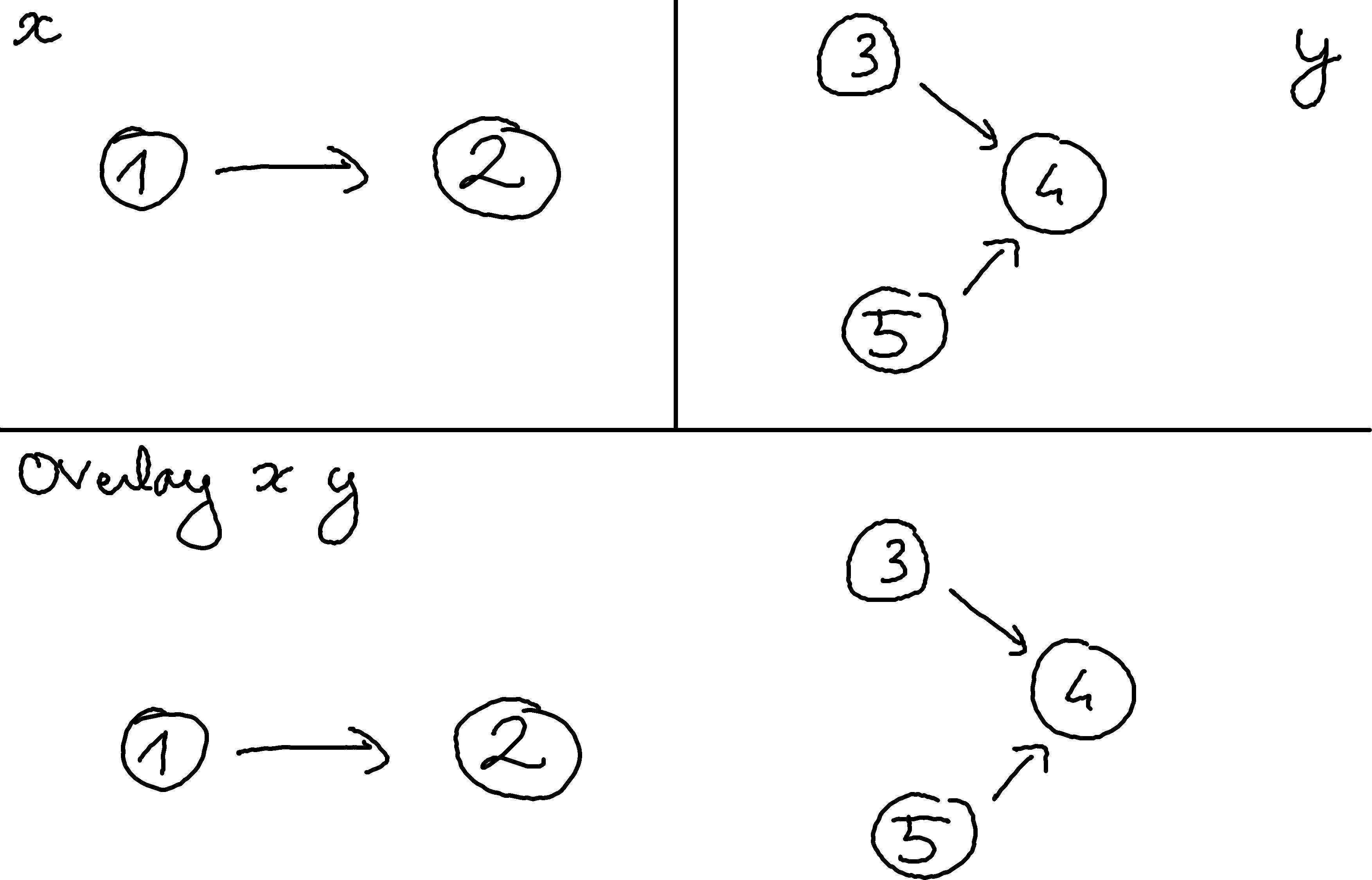4. You can connect two graphs, that is drawing an edge from each vertex of the left side to each vertex to the right side.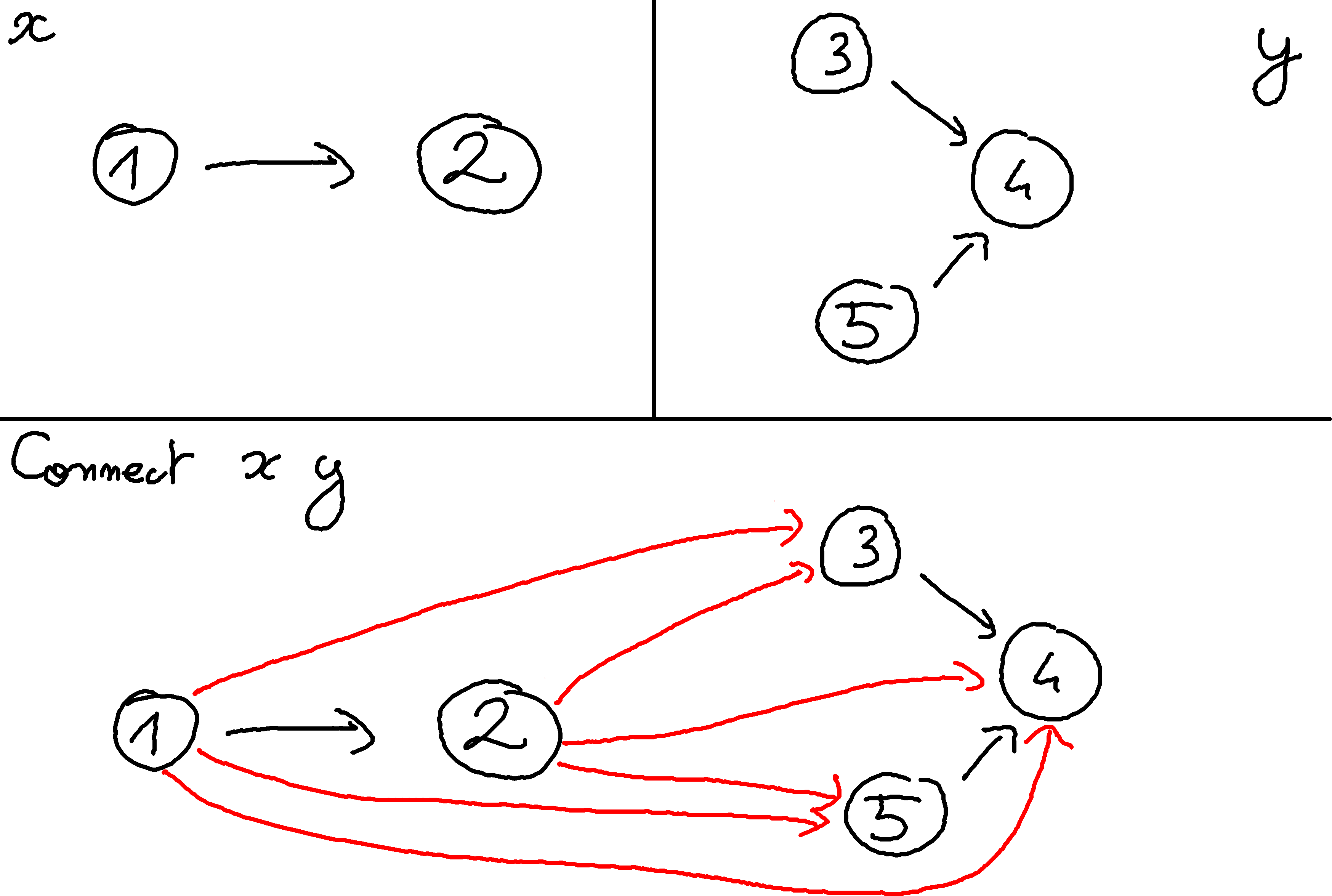Simple, no? … Well ok this is not a standard way to see a graph, but don’t worry, you will get used to it.

Just remember: The only way to create edges is using `Connect`.

This definition allow us to deal with directed graphs: An edge from vertex 1 to vertex 2 is NOT the same than an edge from vertex 2 to vertex 1.

## Some examples

So, how to use this definition? Here is some examples:

• A single path, from a vertex 0 to a vertex 1 can be viewed as `Connect (Vertex 0) (Vertex 1)`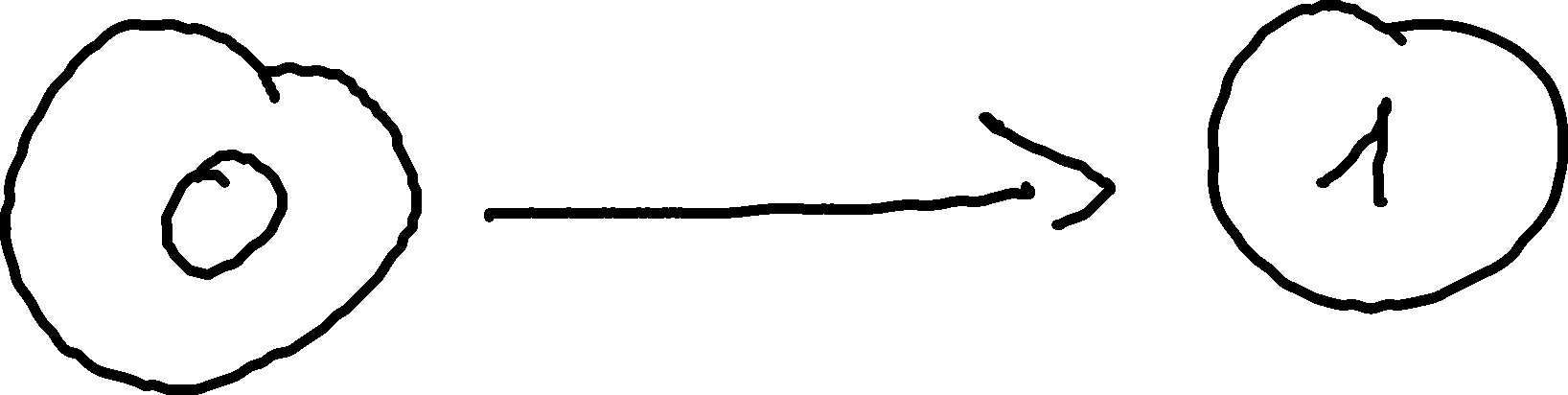• A triangle, with an edge from vertex 0 to vertex 1, an edge from vertex 0 to vertex 2, and an edge from vertex 1 to vertex 2 can be viewed as `Connect (Vertex 0) (Connect (Vertex 1) (Vertex 2))`.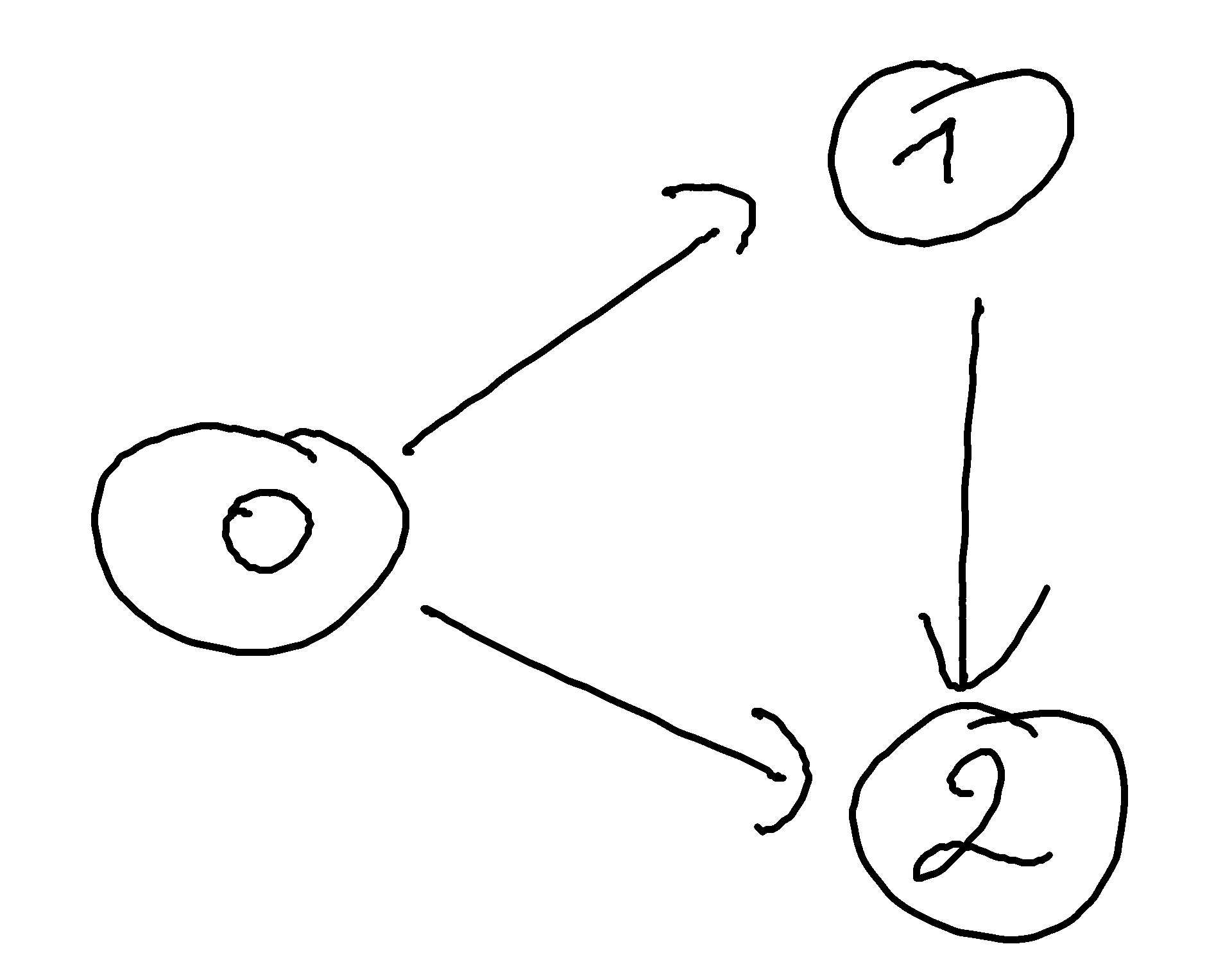I heard you from my desktop:

“Berk, but writing big graphs by hand can become very annoying !“

Don’t worry, there are some shortcuts.

# Going deeper in the definition

## The Num instance

`Overlay` and `Connect` look like operators, and we want to use them as. So we pose:

``````(+) = Overlay
(*) = Connect``````

In fact, if we have something of the `Num` instance, we can transform it directly into a graph using `Vertex`. This leads to this instance:

``````instance Num a => Num (Graph a) where
fromInteger = Vertex . fromInteger
(+)         = Overlay
(*)         = Connect
signum      = const Empty
abs         = id
negate      = id``````

This means that, in a context of a `Graph`, we have `Vertex 1 == 1`, which is quite useful!

Do you see why alga is an implementation of an algebra of graphs? There is a lot of maths here! No please don’t run away like you have seen a zombie in a graveyard! Don’t worry, this is not-so-difficult math.

#### Note

We will use the `(+)` and `(*)` notation, but these laws are true even when dealing with any graphs.

## Overlay

As usual, `(+)` is associative (the order in which you are choosing to overlay graphs is not important):

``(1 + 2) + 3 == 1 + (2 + 3)``

`(+)` is also commutative (overlaying a and b is the same as overlaying b and a):

``1 + 2 == 2 + 1``

`(+)` has `Empty` as a neutral element (overlaying an `Empty` graph to another graph is this graph):

``1 + Empty == 1 == Empty + 1``

`(+)` is idempotent (overlaying a graph with itself is the same graph):

``1 + 1 == 1``

## Connect

As usual, `(*)` is associative (the order in which you are choosing to connect graphs is not important):

``(1 * 2) * 3 == 1 * (2 * 3)``

`(*)` is NOT commutative (drawing an edge from vertex 1 to vertex 2 is not the same as drawing an edge from vertex 2 to vertex 1):

``1 * 2 /= 2 * 1``

`(*)` it has `Empty` as a neutral element (connecting an `Empty` graph to another graph is this graph):

``1 * Empty == 1 == Empty * 1``

`(*)` can saturate (connecting three times the same graph is the same as connecting two times the same graph)

``1 * 1 * 1 == 1 * 1``

Why `(*)` is not idempotent? Because connecting a vertex with himself allow to create a loop: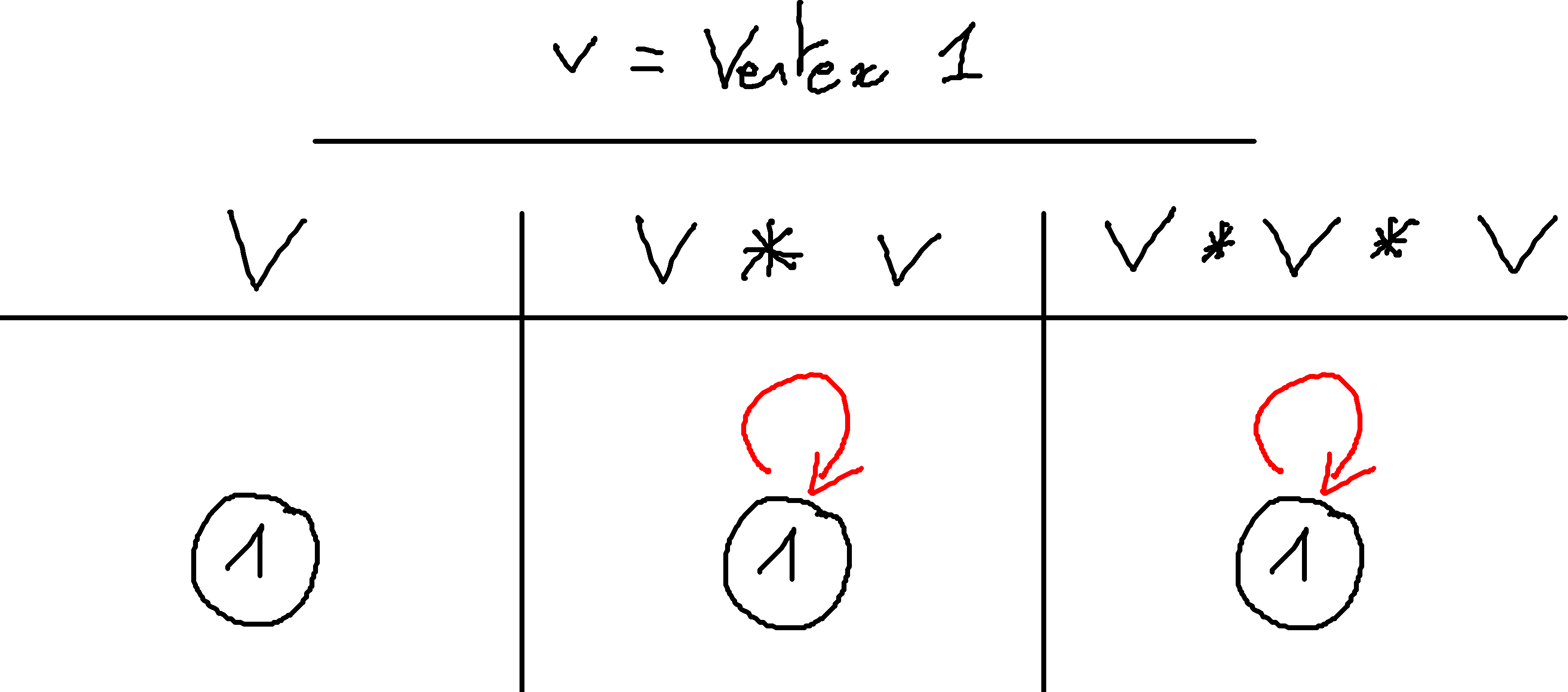## The two together

Do you remind when you have discovered that you can mix + and * in the same equation? This is the same thing here!

``1 * (2 + 3) == 1 * 2 + 1 * 3``

Connecting the single vertex 1 to both 2 and 3 can be done of two equivalent ways:

• either you are connecting 1 to 2 and 3 “overlayed“.

• or you are overlaying the two edges `(1,2)` and `(1,3)`.

Whew, this is done we can make a step forward.

## Making graphs

I haven’t answered on question yet:

Is the definition usable? Can we represent every graph in alga’s representation ?

Let’s try to answer this important question. As said, graphs are (almost all the time) defined as a pair V of vertices and E ⊆ V × V a set of edges. So to prove that we can represents any graph, we need to define a function `create :: [a] -> [(a,a)] -> Graph a` that create a graph from this standard representation.

Let us forget about the edges: we are first going to make `vertices :: [a] -> Graph a` that transform a list of vertices into a `Graph` containing all the single vertices. It looks like we are going to `fold` a list

``````vertices :: [a] -> Graph a
vertices = foldr (\v g -> Overlay (Vertex v) g) Empty``````

Any idea how to do `edges :: [(a,a)] -> Graph a`? The same way, obviously:

``````edges :: [(a,a)] -> Graph a
edges = foldr
(\(x,y) g -> Overlay (Connect (Vertex x) (Vertex y)) g)
Empty``````

And so, what can be our `create :: [a] -> [(a,a)] -> Graph a`? Simply:

``````create :: [a] -> [(a,a)] -> Graph a
create v e = Overlay (vertices v) (edges e)``````

So we have defined the desired function, thus we can safely use the alga’s definition!

# The benefits of the definition

## foldg

One of the very advantage given by this representation is the ability to define the `foldg` function, a kind of adapted `fold` for graph:

``````foldg :: b -> (a -> b) -> (b -> b -> b)
-> (b -> b -> b) -> Graph a -> b
foldg e v o c = go
where
go Empty         = e
go (Vertex  x  ) = v x
go (Overlay x y) = o (go x) (go y)
go (Connect x y) = c (go x) (go y)``````

In other words, the `foldg` function take a base case for `Empty` graphs, something to transform a `Vertex` and combining functions when we encounter `Overlay` or `Connect`.

## transpose

We have a wonderful graph and we want to `transpose` it. Transposing an directed graph consist in inverting the orientation of all edges. Using `foldg`, this is a piece of cake:

``````transpose :: Graph a -> Graph a
transpose = foldg Empty Vertex Overlay (flip Connect)``````

## induce

Still not convinced? Let’s try to build an induced sub-graph. An induced sub-graph is a sub-graph that “forget“ about some vertices and all edges to and from these vertices.

So we are going to code the `induce :: (a -> bool) -> Graph a -> Graph a` function. We will use `foldg` of course.

What is the base case? Do we need to change an `Empty` graph? Obviously, not at all:

``````induce :: (a -> Bool) -> Graph a -> Graph a
induce predicate = foldg
Empty
undefined
undefined
undefined``````

Then if we encounter a vertex, we need to verify if it satisfy the predicate. If it does not, we will simply replace it…Let’s say by the `Empty` graph!

``````induce :: (a -> Bool) -> Graph a -> Graph a
induce predicate = foldg
Empty
(\x -> if predicate x then Vertex x else Empty)
undefined
undefined``````

And finally do we need to touch connection between base graphs? Not at all! Remember, `Empty` is the neutral element of both `Connect` and `Overlay`. So we can leave our empty graphs inside the structure without problem (don’t worry, the real implementation get rid of these empty leaves). So we come to:

``````induce :: (a -> Bool) -> Graph a -> Graph a
induce predicate = foldg
Empty
(\x -> if predicate x then Vertex x else Empty)
Overlay
Connect``````

So simple, isn’t it?

This even allow us to define:

``````removeVertex :: a -> Graph a -> Graph a
removeVertex x = induce (/=x)``````

## hasEdge

`foldg` and `induce` are so cool that a good part of the Alga API is made from them. For example, let’s take a look at the `hasEdge` definition:

``````hasEdge :: Ord a => a -> a -> Graph a -> Graph a
hasEdge u v =
(Connect (Vertex u) (Vertex v) `isSubgraphOf`) .
induce (`elem` [u, v])``````

To check if a graph contains an edge from x to y, you can remove every vertices different of x and y, and then check if the edge alone is a sub-graph of the induced sub-graph. Note that `hasEdge` is requiring an `Ord` instance because `isSubgraphOf` is requiring it.

# The problems of the definition

## Equality

There is no canonical way to define a graph in alga. For example:

``````Overlay (Vertex 1) (Vertex 2)
== Overlay (Vertex 2) (Vertex 1)
== Connect Empty (Overlay (Vertex 1) (Vertex 2))
== Overlay
(Connect (Vertex 1) Empty)
(Connect Empty (Vertex 2))``````

Fortunately, you don’t have to bother with the internal definition since the `Eq` instance (which provide `(==)`) take care of this problem for you.

Alga is also providing `(===)` which denote structural equality, and thus:

``````Overlay (Vertex 1) (Vertex 2)
===
Overlay (Vertex 2) (Vertex 1)
== False``````

## Take care when defining functions

Here is a nasty function that you can define:

``````close : Graph A -> Graph A
close Empty         = Empty
close (Vertex x)    = Vertex x
close (Overlay x y) = Connect x y
close (Connect x y) = Connect x y``````

Do you see the problem?

``````>>> let x = Vertex 0
>>> let y = Overlay (Vertex 0) (Vertex 0)
>>> x == y
True
>>> print (close x)
Vertex 0
>>> print (close y)
(Vertex 0) * (Vertex 0)
>>> close x == close y
False``````

For the moment, one can mess the internal structure, and the equality loose its meaning (ie ∀(f:Graph AGraph B) : g = y ⟹ f g = f y does NOT hold ).

# Useful instances

Alga’s graphs are instance of some classical Haskell classes:

## Eq, Show

Of course, you have Graph equality, and you can show a Graph. Alga can also export to the DOT file format through the `Algebra.Graph.Export.Dot` module.

## Ord

Less trivially, there is a total order defined on graphs and implemented in alga. It use the size-lexicographic/ comparison:

• Compare the number of vertices. In case of a tie, continue.

• Compare the sets of vertices. In case of a tie, continue.

• Compare the number of edges. In case of a tie, continue.

• Compare the sets of edges.

So first, it is indeed and order (this relation is transitive, reflexive and anti-symmetric) and it is total (you can compare any graphs). The second is that this order is, in some way, compatible with graphs operations:

• g: `(empty <= x) == True`

• If x is a sub-graph of y, then `(x <= y) == True`

• x, y: `(x <= x+y) == True`

• x, y: `(x+y <= x*y) == True`

## Functor

Not so surprisingly, `Graph` is an instance of `Functor`:

``````instance Functor (Graph a) where
fmap _ Empty = Empty
fmap f (Vertex a) = Vertex \$ f a
fmap f (Overlay a b) = Overlay (fmap f a) (fmap f b)
fmap f (Connect a b) = Connect (fmap f a) (fmap f b)``````

This means that if you have something to transform a `a` in a `b` then you can transform a `Graph a` into a `Graph b`. For example: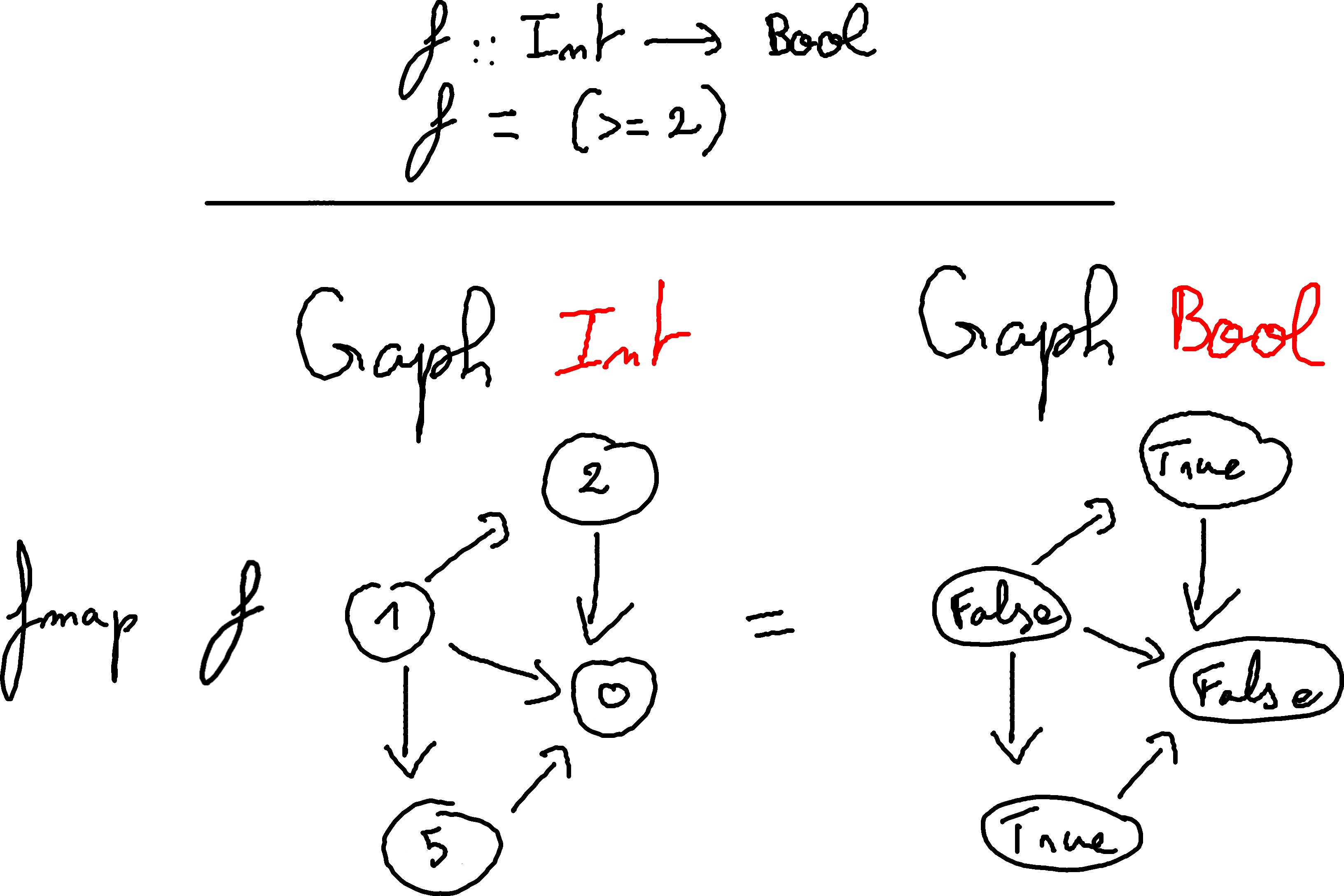If you want to test it, the first graph in alga’s representation is: `1 * (2 + 5) * 0`

Alert! Alert! Haskeller’s alarms are ringing! If there is a `Functor` instance, is there a `Monad` one?

`Graph` are indeed a `Monad` instance:

``````instance Monad (Graph a) where
return  = Vertex
g >>= f = foldg Empty f Connect Overlay g``````

You can convert anything into a graph, simply by transforming it in a single vertex. Moreover, if you can produce a graph from a type `a` then you can replace every vertex of a `Graph a` with the result, transforming it into a `Graph b`.

For example, one can redefine the previously-viewed `induce` as:

``````induce :: (a -> Bool) -> Graph a -> Graph a
induce predicate g
= g >>= (\x -> if predicate x then Vertex x else Empty)``````

## Foldable and Traversable

`Graph` can be a valid `Foldable` and a valid `Traversable` instance, but these are not defined in the `Algebra.Graph` module.
The reason is that these instances are not compatible with the rest of the library. For example, `vertexList g /= toList g` because a vertex can be multiple times in the structure.

# An example: A social network

## The goal

Ok, now we are wanting to build something real with all of this. Let’s say a social network: one can represent them easily through graphs. The marketing team analysed the market, and decided to make something “à la Twitter“. The vertices will be users, and an edge from x to y will denote that x is following y.

## handleRequest

The staff meeting has chosen you to build the `handleRequest` function:

``````type User = Int

| RemoveUser User
| ConnectU User User
| DisconnectU User User

handleRequestM :: RequestM -> Graph User -> Graph User``````

This is now a pretty simple job and the implementation is straightforward:

``````handleRequestM :: RequestM -> Graph User -> Graph User
handleRequestM (AddUser a) = Overlay (Vertex a)
handleRequestM (RemoveUser a) = removeVertex a
handleRequestM (ConnectU a b) =
Overlay (Connect (Vertex a) (Vertex b))
handleRequestM (DisconnectU a b) = removeEdge a b``````

## Inspection

Viewing that you implemented your function very quickly, you are being asked to help one of your co-workers on his function. He was working about the `getFollowing :: User -> Graph User -> [User]` function.

One possible way is to use the `edgeList :: Ord a => Graph a -> [(a,a)]` function.

``````getFollowing :: User -> Graph User -> [User]
getFollowing u =
map snd . filter (\(v,_) -> u == v ) . edgeList``````

you can even implement blindly the `getFollowers` function:

``````getFollowers :: User -> Graph User -> [User]
getFollowers u =
map fst . filter (\(_,v) -> u == v ) . edgeList``````

## Going IO

Ok, pure `Graph` inspection is cool, but how do inspect with IO? Your superior want to know from time to time how many users are connected. He has wrote `isConnected` `:: User -> IO Bool`, and he is asking you to write `numberOfConnected` `:: Graph User -> IO Int`. Using `traverse` on the list of the vertices, you quickly answer:

``````numberOfConnected :: Graph User -> IO Int
numberOfConnected = fmap (length . filter id) .
foldg (pure Empty) (fmap Vertex . isConnected) (liftA2 Overlay) (liftA2 Connect) .
vertexList``````

Note that this version is easy to understand and to write, but nor very efficient. One can write a more efficient one using `foldg` and `IntSet`:

``````import qualified Data.IntSet as Set
import Control.Applicative (liftA2)

numberOfConnected :: Graph User -> IO Int
numberOfConnected = fmap Set.size . foldg
(return Set.empty)
(\x -> fmap
(\y -> if y
then Set.singleton x
else Set.empty
)
(isConnected x)
)
(liftA2 Set.union)
(liftA2 Set.union)``````